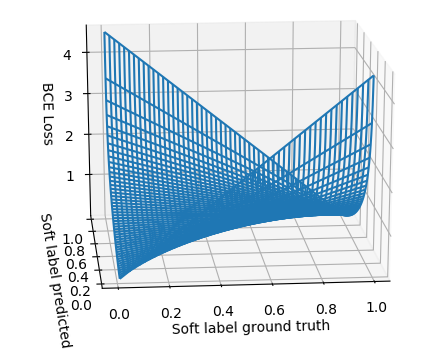# Cross entropy for soft label

The cross entropy in pythorch can’t be used for the case when the target is soft label, a value between 0 and 1 instead of 0 or 1.

I code my own cross entropy, but i found the classification accuracy is always worse than the nn.CrossEntropyLoss() when i test on the dataset with hard labels, here is my loss:

``````class softCrossEntropy(nn.Module):
def __init__(self):
super(softCrossEntropy, self).__init__()
return

def forward(self, inputs, target):
"""
:param inputs: predictions
:param target: target labels
:return: loss
"""
log_likelihood = - F.log_softmax(inputs, dim=1)
sample_num, class_num = target.shape
loss = torch.sum(torch.mul(log_likelihood, target))/sample_num

return loss
``````

could anyone help me to check if there is problem in my code?

2 Likes

Note that cross-entropy for non 0/1 labels is not symmetric, which could be an explanation for the poor performance.

E.g., consider the scenario for the binary cross entropy:Or consider the following, where the ground truth and the predicted labels are shown on the x axis. I.e., you can see that if both are 0, the cost is zero. However, if both are 0.5, the cost is almost 0.7, although prediction=true label10 Likes

similar with the label smoothing:

I believe this method from the new Snorkel lib is what you want: